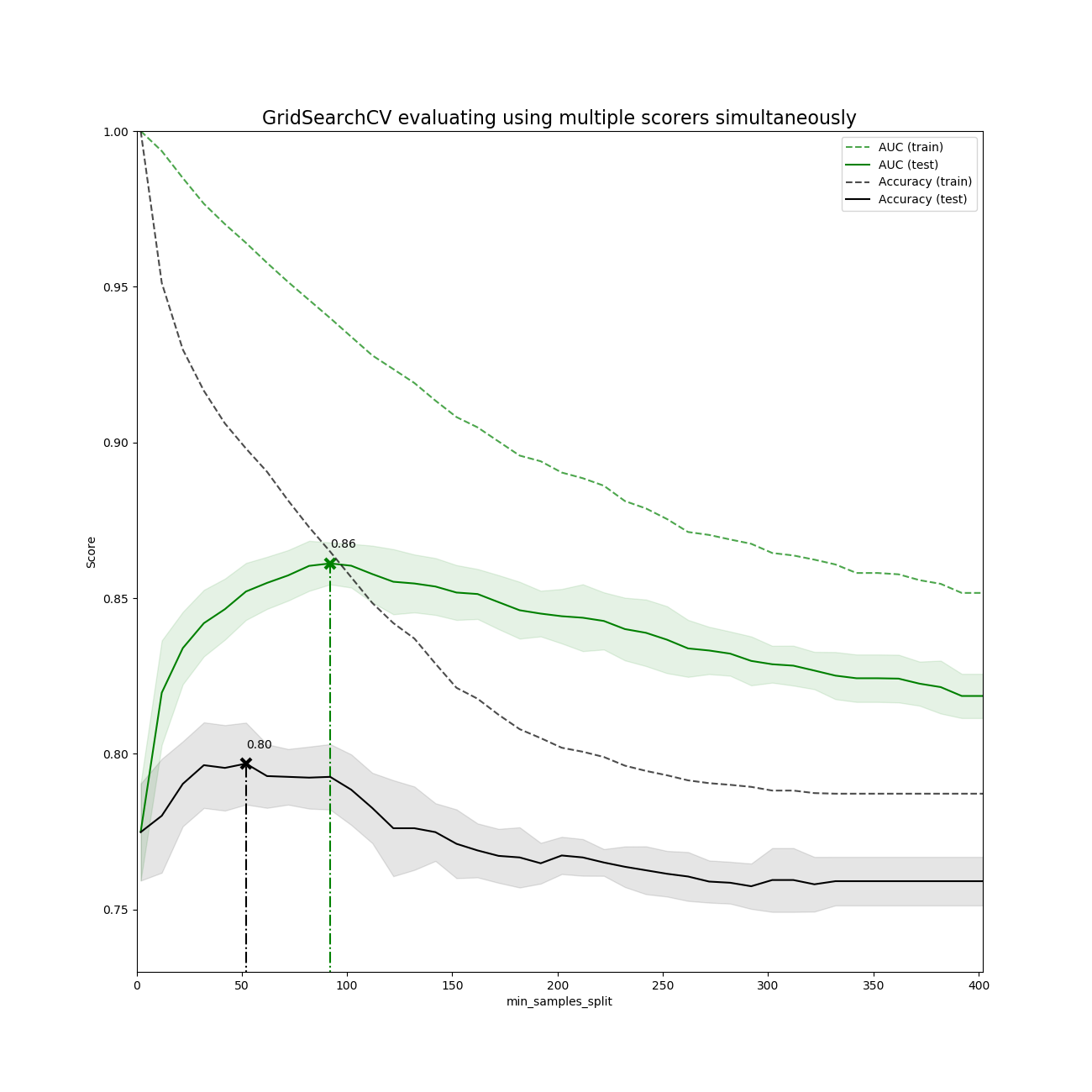# Demonstration of multi-metric evaluation on cross_val_score and GridSearchCV¶

Multiple metric parameter search can be done by setting the `scoring` parameter to a list of metric scorer names or a dict mapping the scorer names to the scorer callables.

The scores of all the scorers are available in the `cv_results_` dict at keys ending in `'_<scorer_name>'` (`'mean_test_precision'`, `'rank_test_precision'`, etc…)

The `best_estimator_`, `best_index_`, `best_score_` and `best_params_` correspond to the scorer (key) that is set to the `refit` attribute.

```# Author: Raghav RV <rvraghav93@gmail.com>

import numpy as np
from matplotlib import pyplot as plt

from sklearn.datasets import make_hastie_10_2
from sklearn.model_selection import GridSearchCV
from sklearn.metrics import make_scorer
from sklearn.metrics import accuracy_score
from sklearn.tree import DecisionTreeClassifier

print(__doc__)
```

## Running `GridSearchCV` using multiple evaluation metrics¶

```X, y = make_hastie_10_2(n_samples=8000, random_state=42)

# The scorers can be either be one of the predefined metric strings or a scorer
# callable, like the one returned by make_scorer
scoring = {'AUC': 'roc_auc', 'Accuracy': make_scorer(accuracy_score)}

# Setting refit='AUC', refits an estimator on the whole dataset with the
# parameter setting that has the best cross-validated AUC score.
# That estimator is made available at ``gs.best_estimator_`` along with
# parameters like ``gs.best_score_``, ``gs.best_parameters_`` and
# ``gs.best_index_``
gs = GridSearchCV(DecisionTreeClassifier(random_state=42),
param_grid={'min_samples_split': range(2, 403, 10)},
scoring=scoring, cv=5, refit='AUC')
gs.fit(X, y)
results = gs.cv_results_
```

## Plotting the result¶

```plt.figure(figsize=(13, 13))
plt.title("GridSearchCV evaluating using multiple scorers simultaneously",
fontsize=16)

plt.xlabel("min_samples_split")
plt.ylabel("Score")
plt.grid()

ax = plt.axes()
ax.set_xlim(0, 402)
ax.set_ylim(0.73, 1)

# Get the regular numpy array from the MaskedArray
X_axis = np.array(results['param_min_samples_split'].data, dtype=float)

for scorer, color in zip(sorted(scoring), ['g', 'k']):
for sample, style in (('train', '--'), ('test', '-')):
sample_score_mean = results['mean_%s_%s' % (sample, scorer)]
sample_score_std = results['std_%s_%s' % (sample, scorer)]
ax.fill_between(X_axis, sample_score_mean - sample_score_std,
sample_score_mean + sample_score_std,
alpha=0.1 if sample == 'test' else 0, color=color)
ax.plot(X_axis, sample_score_mean, style, color=color,
alpha=1 if sample == 'test' else 0.7,
label="%s (%s)" % (scorer, sample))

best_index = np.nonzero(results['rank_test_%s' % scorer] == 1)
best_score = results['mean_test_%s' % scorer][best_index]

# Plot a dotted vertical line at the best score for that scorer marked by x
ax.plot([X_axis[best_index], ] * 2, [0, best_score],
linestyle='-.', color=color, marker='x', markeredgewidth=3, ms=8)

# Annotate the best score for that scorer
ax.annotate("%0.2f" % best_score,
(X_axis[best_index], best_score + 0.005))

plt.legend(loc="best")
plt.grid('off')
plt.show()
```Total running time of the script: ( 0 minutes 34.529 seconds)

Generated by Sphinx-Gallery